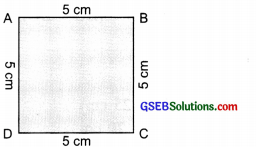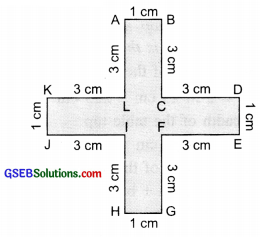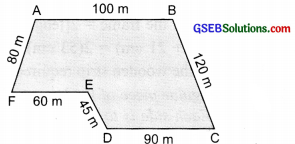# GSEB Solutions Class 6 Maths Chapter 10 Mensuration InText Questions

Gujarat Board GSEB Textbook Solutions Class 6 Maths Chapter 10 Mensuration InText Questions and Answers.

## Gujarat Board Textbook Solutions Class 6 Maths Chapter 10 Mensuration InText Questions

Try These (Page 206)

Question 1.
Measure and write the length of the four sides of the top of your study table.
AB = ________ cm
BC = ________ cm
CD = ________ cm
DA = ________ cmNow, the sum of the lengths of the four sides = AB + BC + CD + DA
= ________ cm + ________ cm + _______ cm + _______ cm = _______ cm
What is the perimeter of the page?
Solution:
AB = 140 cm,
BC = 80 cm,
CD = 140 cm,
DA = 80 cm
∴ AB + BC + CD + DA
= 140 cm + 80 cm + 140 cm + 80 cm = 440 cm
The perimeter = 440 cm
Note: This is an activity so the measures of AB,BC,CD and DA will be different for different table tops.Question 2.
Measure and write the lengths of the four sides of a page of your notebook. The sum of the lengths of the four sides
= AB + BC + CD + DA
= _________ cm + _________ cm + _________ cm + _________ cm = _________ cm
What is the perimeter of the page?
Solution:Question 3.
Meera went to a park 150 m long and 80 m wide. She took one complete round on its boundary. What is the distance covered by her?
Solution:
Length of the park = 150 m
The breadth of the park = 80 m
The perimeter of the park
= 150 m + 80 m + 150 m + 80 m = 460 m
∴ Distance covered by Meera in going one complete round of the park = 460 m.Question 4.
Find the perimeter of the following figures:
(a) Perimeter = AB + BC + CD + DA = ________ + ________ + ________ + = ________(b) Perimeter = AB + BC + CD + DA = ________ + ________ + ________ + = ________(c) Perimeter = AB + BC + CD + DE + EF + FG + GH + HI + IJ + JK + KL + LA
= ________ + ________ + ________ + ________ + ________ + ________ + ________ + ________ + ________ + ________ + ________ + ________ = ________(d) Perimeter = AB + BC + CD + DE + EF + FA________ + ________ + ________ + ________ + ________ + ________ = ________Note: For finding the perimeter of any closed figure made up entirely of the line segment, simply find the sum of the lengths of all the sides.
Solution:
(a) ∵ AB = 40 cm, BC = 10 cm,
AD = 10 cm and DC = 40 cm
∴ Perimeter = AB + BC + AD + DC
= 40 cm + 10 cm + 10 cm + 40 cm = 100 cm

(b) ∵ AB = 5 cm, BC = 5 cm,
CD = 5 cm and AD = 5 cm
∴ Perimeter = AB + BC + CD + AD
= 5 cm + 5 cm + 5 cm + 5 cm = 20 cm

(c) Here, perimeter = AB + BC + CD + DE + EF + FG + GH + HI + IJ + JK + KL + LA
= 1 cm + 3 cm + 3 cm + 1 cm + 3 cm
+ 3 cm + 1 cm + 3 cm + 3 cm + 1 cm
+ 3 cm + 3 cm = 28 cm

(d) Here, perimeter=AB + BC + CD + DE + EF + FA
= 100 m + 120 m + 90 m + 45 m + 60 m + 80 m = 495 mTry These (Page 208)

Question 1.
Find the perimeter of the following rectangles:Solution:
Do yourself.Try These (Page 211)

Question 1.
Find various objects from your surroundings that have regular shapes and find their perimeters.
Solution:
It is project work. You can find objects having regular shapes such as a ‘nameplate’ a ‘road signal’, etc. Measure their sides and find perimeters.Try These (Page 215)

Question 1.
Draw any circle on a graph sheet. Count the squares and use them to estimate the area of the circular region.
Solution:
It is an ‘activity’. Please do it yourself.

Question 2.
Trace shapes of leaves, flower petals, and other such objects on the graph paper and find their areas.
Solution:
It is an ‘activity’. Please do it yourself.Try These (Page 217)

Question 1.
Find the area on the floor of your classroom.
Solution:
This is an activity. Please do it yourself.

Question 2.
Find the area of anyone’s door in your house.
Solution:
This is an activity. Please do it yourself.